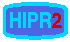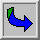# Histogram Equalization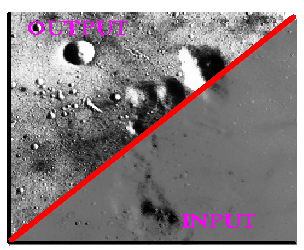Common Names: Histogram Modeling, Histogram Equalization

## Brief DescriptionHistogram modeling techniques (e.g. histogram equalization) provide a sophisticated method for modifying the dynamic range and contrast of an image by altering that image such that its intensity histogram has a desired shape. Unlike contrast stretching, histogram modeling operators may employ non-linear and non-monotonic transfer functions to map between pixel intensity values in the input and output images. Histogram equalization employs a monotonic, non-linear mapping which re-assigns the intensity values of pixels in the input image such that the output image contains a uniform distribution of intensities (i.e. a flat histogram). This technique is used in image comparison processes (because it is effective in detail enhancement) and in the correction of non-linear effects introduced by, say, a digitizer or display system.## How It Works

Histogram modeling is usually introduced using continuous, rather than discrete, process functions. Therefore, we suppose that the images of interest contain continuous intensity levels (in the interval [0,1]) and that the transformation function f which maps an input image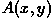onto an output image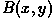is continuous within this interval. Further, it will be assumed that the transfer law (which may also be written in terms of intensity density levels, e.g.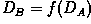) is single-valued and monotonically increasing (as is the case in histogram equalization) so that it is possible to define the inverse law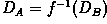. An example of such a transfer function is illustrated in Figure 1.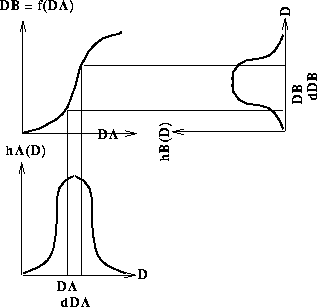Figure 1 A histogram transformation function.

All pixels in the input image with densities in the region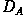to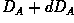will have their pixel values re-assigned such that they assume an output pixel density value in the range from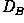to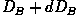. The surface areasand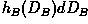will therefore be equal, yielding: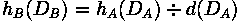where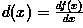.

This result can be written in the language of probability theory if the histogram h is regarded as a continuous probability density function p describing the distribution of the (assumed random) intensity levels: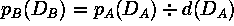In the case of histogram equalization, the output probability densities should all be an equal fraction of the maximum number of intensity levels in the input image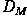(where the minimum level considered is 0). Thetransfer function (or point operator) necessary to achieve this result is simply:Therefore,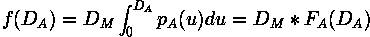where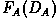is simply the cumulative probability distribution (i.e. cumulative histogram) of the original image. Thus, an image which is transformed using its cumulative histogram yields an output histogram which is flat!

A digital implementation of histogram equalization is usually performed by defining a transfer function of the form: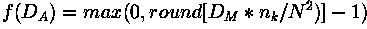where N is the number of image pixels and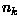is the number of pixels at intensity level k or less.

In the digital implementation, the output image will not necessarily be fully equalized and there may be `holes' in the histogram (i.e. unused intensity levels). These effects are likely to decrease as the number of pixels and intensity quantization levels in the input image are increased.## Guidelines for Use

To illustrate the utility of histogram equalization, consider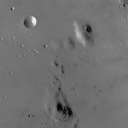which shows an 8-bit grayscale image of the surface of the moon. The histogram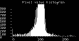confirms what we can see by visual inspection: this image has poor dynamic range. (Note that we can view this histogram as a description of pixel probability densities by simply scaling the vertical axis by the total number of image pixels and normalizing the horizontal axis using the number of intensity density levels (i.e. 256). However, the shape of the distribution will be the same in either case.)

In order to improve the contrast of this image, without affecting the structure (i.e. geometry) of the information contained therein, we can apply the histogram equalization operator. The resulting image isand its histogram is shown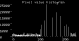Note that the histogram is not flat (as in the examples from the continuous case) but that the dynamic range and contrast have been enhanced. Note also that when equalizing images with narrow histograms and relatively few gray levels, increasing the dynamic range has the adverse effect of increasing visual grainyness. Compare this result with that produced by the linear contrast stretching operatorIn order to further explore the transformation defined by the histogram equalization operator, consider the image of the Scott Monument in Edinburgh, ScotlandAlthough the contrast on the building is acceptable, the sky region is represented almost entirely by light pixels. This causes most histogram pixels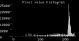to be pushed into a narrow peak in the upper graylevel region. The histogram equalization operator defines a mapping based on the cumulative histogram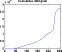which results in the imageWhile histogram equalization has enhanced the contrast of the sky regions in the image, the picture now looks artificial because there is very little variety in the middle graylevel range. This occurs because the transfer function is based on the shallow slope of the cumulative histogram in the middle graylevel regions (i.e. intensity density levels 100 - 230) and causes many pixels from this region in the original image to be mapped to similar graylevels in the output image.

We can improve on this if we define a mapping based on a sub-section of the image which contains a better distribution of intensity densities from the low and middle range graylevels. If we crop the image so as to isolate a region which contains more building than sky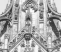we can then define a histogram equalization mapping for the whole image based on the cumulative histogram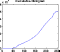of this smaller region. Since the cropped image contains a more even distribution of dark and light pixels, the slope of the transfer function is steeper and smoother, and the contrast of the resulting imageis more natural. This idea of defining mappings based upon particular sub-sections of the image is taken up by another class of operators which perform Local Enhancements as discussed below.

## Common VariantsHistogram Specification

Histogram equalization is limited in that it is capable of producing only one result: an image with a uniform intensity distribution. Sometimes it is desirable to be able to control the shape of the output histogram in order to highlight certain intensity levels in an image. This can be accomplished by the histogram specialization operator which maps a given intensity distribution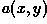into a desired distribution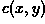using a histogram equalized image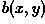as an intermediate stage.

The first step in histogram specialization, is to specify the desired output density function and write a transformation g(c). If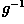is single-valued (which is true when there are no unfilled levels in the specified histogram or errors in the process of rounding offto the nearest intensity level), thendefines a mapping from the equalized levels of the original image,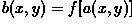. It is possible to combine these two transformations such that the image need not be histogram equalized explicitly: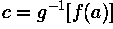Local Enhancements

The histogram processing methods discussed above are global in the sense that they apply a transformation function whose form is based on the intensity level distribution of an entire image. Although this method can enhance the overall contrast and dynamic range of an image (thereby making certain details more visible), there are cases in which enhancement of details over small areas (i.e. areas whose total pixel contribution to the total number of image pixels has a negligible influence on the global transform) is desired. The solution in these cases is to derive a transformation based upon the intensity distribution in the local neighborhood of every pixel in the image.

The histogram processes described above can be adapted for local enhancement. The procedure involves defining a neighborhood around each pixel and, using the histogram characteristics of this neighborhood, to derive a transfer function which maps that pixel into an output intensity level. This is performed for each pixel in the image. (Since moving across rows or down columns only adds one new pixel to the local histogram, updating the histogram from the previous calculation with new data introduced at each motion is possible.) Local enhancement may also define transforms based on pixel attributes other than histogram, e.g. intensity mean (to control variance) and variance (to control contrast) are common.

## Interactive Experimentation

You can interactively experiment with this operator by clicking here.

## Exercises

1. Suppose that you have a 128×128 square pixel image with an 8 gray level intensity range, within which the lighter intensity levels predominate as shown in the table below. A) Sketch the histogram (number of pixels vs gray level) to describe this distribution. B) How many pixels/gray levels would there be in an equalized version of this histogram? C) Apply the discrete transformation described above and plot the new (equalized) histogram. (How well does the histogram approximate a uniform distribution of intensity values?)

``` -------------------------------
| Gray Level | Number of Pixels |
|------------+------------------|
| 0          | 34               |
|------------+------------------|
| 1          | 50               |
|------------+------------------|
| 2          | 500              |
|------------+------------------|
| 3          | 1500             |
|------------+------------------|
| 4          | 2700             |
|------------+------------------|
| 5          | 4500             |
|------------+------------------|
| 6          | 4000             |
|------------+------------------|
| 7          | 3100             |
-------------------------------```

2. Suppose you have equalized an image once. Show that a second pass of histogram equalization will produce exactly the same result as the first.

3. Interpreting images derived by means of a non-monotonic or non-continuous mapping can be difficult. Describe the effects of the following transfer functions:

(a) f has a horizontal plateau,

(b) f contains a vertical jump,

(c) f has a negative slope.

(Hint: it can be useful to sketch the curve, as in Figure 1, and then map a few points from histogram A to histogram B.)

4. Apply local histogram equalization to the imageCompare this result with those derived by means of the global transfer function shown in the above examples.

5. Apply global and local histogram equalization to the montage image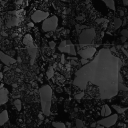## References

R. Boyle and R. Thomas Computer Vision: A First Course, Blackwell Scientific Publications, 1988, pp 35 - 41.

R. Gonzalez and R. Woods Digital Image Processing, Addison-Wesley Publishing Company, 1992, Chap. 4.

A. Jain Fundamentals of Digital Image Processing, Prentice-Hall, 1986, pp 241 - 243.

A. Marion An Introduction to Image Processing, Chapman and Hall, 1991, Chap. 6.

## Local Information